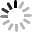Home News

## How to calculate the Gas Spring Force ?

I'm Online Chat Now
Company News
How to calculate the Gas Spring Force ?

1 – Gas spring pressure/force

The pressure required for the gas spring is calculated by substituting the values used in the following formula:

F1 = ((m x RH)/(2 x N x x2) + 5) x 9.81 = gas spring pressure expressed in Newtons

• F1 = gas spring pressure/force
• N = number of gas springs desired
• m = weight (kg) of the tailgate/object to be lifted, manoeuvred
• RH = length (in metres) of the tailgate object to be lifted, manoeuvred
• x2 = distance (in metres) between the point of application of the gas spring on the tailgate and the rotation axis of the tailgate.

The number 5 represents a constant force of 5 kg/p, equivalent to rubbing actions.

Example:

For a hatchback with the following characteristics and values:

• N = 2
• m = 50 kg
• RH = 1,50 m
• x2 = 0.40 m

F1 = ((50 x 1.5) / (2 × 2 × 0.40) + 5) = 9.81 x 508N

In this case, since the tolerance is +/- 50 N, we would recommend two 550N gas springs.Pub Time : 2017-02-16 15:13:15
Contact Details
Guangzhou Automotive Co., Ltd.

Contact Person: Mr. Jerry Ou

Tel: +86-13539882798

Send your inquiry directly to us (0 / 3000)